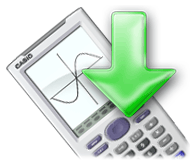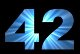# Programing Cp 330

2 replies to this topic

### #1hadi irani

Newbie

•• Members
•• 3 posts
• Gender:Male
• Location:iRan
• Interests:whatching movies & series,...

• Calculators:

Posted 15 December 2012 - 12:10 PM

hi guys
can you help me?

step 1 : get parameter "C,B,d,Fc,As,d',A's" from user
and calculate this number solve
F's=700(1-d'/c)
Fs=700(d/c-1)

step 2 : if Fs<Fy continue, else input new "C"

step 3 : calculate a=b*C next => P=a*0.65*Fc*(a*B-A's)+A's*0.85*F's-As*0.85*Fs
a:alfa
b:beta

step 4: e'=(1/p)*[alfa*.65*Fc*(a*B-A's)(d-a/2)+A's*.85*F's*(d-d')]

step 5 : comparison e' with e0'
e0'~=e' : nip-and-tuck
e'>e0' : again step 1 and choose bigger "C"
e'<e0' : return step 1 and choose smaller "C"
ps: maybe Fs become negative, input that
alfa= .85-.0015*Fc
beta= .97-.0025*Fc
e0'= e+d''
i want get data from user and next calculate then give out F's, P, e'

Edited by hadi irani, 15 December 2012 - 12:28 PM.

### #2Forty-Two

Forty-Two

Casio Overlord

•• Deputy
•• 528 posts
• Gender:Male
• Location:Well, The sign says "You are here"...

• Calculators:
Casio fx-CG10 Prizm
Casio fx-9860GII
TI-84+ SE

Posted 16 December 2012 - 03:47 PM

Have you looked in the user's manual? They usually have lots of information on how to program in basic.

### #3MicroPro

MicroPro

Casio Overlord

•• Deputy
•• 640 posts
• Gender:Male
• Location:Iran

• Calculators:

Posted 30 December 2012 - 12:12 PM

Beginning can always be difficult. For

Are these differential equations or just numeric variables? If they are simple numeric variables, this can be solved like the following.

You should enter B,d,Fc,As,d_,A_s as parameters in the program editor (In front of the program name and the letter "N").

```Local F_s,Fs

'Step 1 and 2
Do
Input C
700(1-d_/C)⇒F_s
700(d/C-1)⇒Fs
LpWhile not(Fs<Fy)

Lbl Start:
700(1-d_/C)⇒F_s
700(d/C-1)⇒Fs
.85-.0015*Fc⇒alfa
.97-.0025*Fc⇒beta
e+d''⇒e0'

'Step 3
B*C ...⇒a
Alpha * 0.65 .... ⇒P

'Step 4
(1.p)*(alfa * 0.65 * Fc ...)⇒e_

'Step 5
If abs(e0_ - e_) < 0.001 : Then
Print "Almost equal"
Print F_s
Print P
Print e_
ElseIf e_ > e0_ : Then
C+1 ⇒ C
Goto Start
ElseIf e_ < e0_ : Then
C-1 ⇒ C
Goto Start
IfEnd
```

Note: Because the character ' (prime) is not allowed, I used _ in variable names instead. And I didn't write the complete formulas in the above code.

Where did Fy and d'' come from? Their value should be set somewhere.

I was not sure where you want the F_s and P and e' displayed, so put them in the "e0'~=e" part.